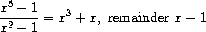# Division of univariate polynomial

A univariate polynomial can be divided by another one to produce a polynomial quotient and a polynomial remainder. For example,Division can be performed via long division. That is, divide the highest-order term of the dividend by the highest-order term of the divisor. The result is the first term of the quotient. Next, multiply the result by the divisor, subtract that from the dividend, and produce the rest of the answer by recursively dividing the difference by the divisor. Stop when the order of the divisor exceeds the order of the dividend and declare the dividend to be the remainder. Also, if the dividend ever becomes zero, return zero as both quotient and remainder.

We can design a `div-poly` procedure on the model of `add-poly` and `mul-poly` . The procedure checks to see if the two polys have the same variable. If so, `div-poly` strips off the variable and passes the problem to `div-terms` , which performs the division operation on term lists. `div-poly` finally reattaches the variable to the result supplied by `div-terms` . It is convenient to design `div-terms` to compute both the quotient and the remainder of a division. Div-terms can take two term lists as arguments and return a list of the quotient term list and the remainder term list.

Complete the following definition of `div-terms` by filling in the missing expressions. Use this to implement `div-poly` , which takes two `poly` as arguments and returns a list of the `poly` quotient and `poly` remainder.

``````(define (div-terms L1 L2)
(if (empty-termlist? L1)
(list (the-empty-termlist) (the-empty-termlist))
(let ((t1 (first-term L1))
(t2 (first-term L2)))
(if (> (order t2) (order t1))
(list (the-empty-termlist) L1)
(let ((new-c (div (coeff t1) (coeff t2)))
(new-o (- (order t1) (order t2))))
(let ((rest-of-result
<compute rest of result recursively>
))
<form complete result>
))))))
``````